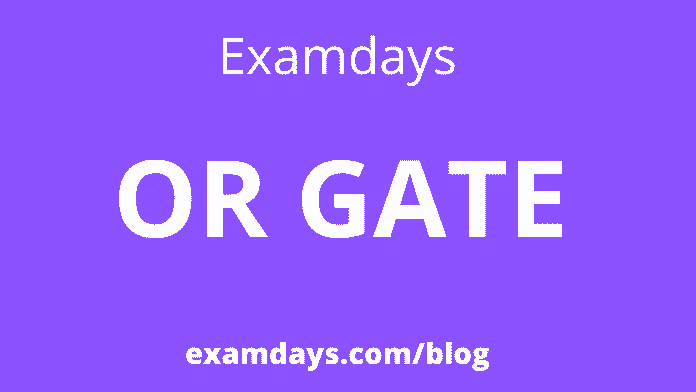# OR Gate Truth Table Circuit Diagram Formula IC Number

0Logic gates are an important topic if you are studying electronics. These are essential digital devices based mainly on Boolean functions. Logic gates perform logical operations on single or multiple binary inputs and give a binary output. Simply put, logic gates are electronic circuits in a digital system.

Those who are looking for the OR gate information like Diagram, truth table, IC number, Further details are added on this page for the candidate’s reference purpose.

## OR GATE

OR gate is a widely used digital logic circuit. The OR gate output state is always low when both inputs are low. If any input value of an OR gate is set to 1, it will always produce a high-level output (1). The OR gate gets its name because it behaves after a logical inclusive “or” fashion. Output is “true” if one or both inputs are “true”. If both inputs are “false”, the output is “false”. In other words, for the output to be 1, at least one or both inputs must be 1

## OR Gate Truth Table

An OR gate has an output signal with two or more inputs. It is called an OR gate because the output signal is high only if any or all of the input signals are high.

## Types of OR Gate

It is divided into three types based on inputs provided to the OR gate. They are

1. Two Inputs OR gate

The two OR gate is an electronic circuit that gives a high output (1) if two of its inputs are high.  A plus symbol (+) is used to show the OR operation.

Three Inputs OR gate

OR gate can have any number of individual inputs. The Boolean expression of a logical OR gate is defined as the binary operation plus(+). Like an AND gate, an OR gate can be cascaded together to form any number of individual inputs. The truth table is given below:

Logical OR gate circuit diagram:

3-input OR Gate

### Multiple-inputs OR Gate

The key points of OR gate:

• An OR gate does logical OR(addition) operations. It has one output and any number of input probes.
• An OR gate uses transistors and operates when both inputs are at logic low.
• When both inputs are high at logic, both transistors are in a saturation state and serve as a short circuit. Hence the output is high if either input is at logic high.
• If both inputs are logic high, the output is high. If both inputs are logic low, the output is low.
• OR gate is a logic gate that executes logical disjunction.
• The diode circuit of the OR gate works by becoming forward-biased and short-circuiting when one of the inputs is given with logic high.

Comparison Between AND Gate vs OR Gate:

What are the differences between AND gate and OR gate?

• AND gate gives a ‘true’ output only when both inputs are ‘true’, whereas OR gate results in an output of ‘true’ if at least one of the inputs is ‘true’.
• AND gate implements logical conjunction, and OR gate implements logical disjunction.

What is Exclusive-OR?

An exclusive-OR gate or XOR gate is achieved by combining standard logic gates. The XOR gate is widely used in error detection circuits, computational logic comparators, and arithmetic circuits. An exclusive OR gate gives an output only if its two inputs are unequal, that is, if one is high (one) and the other is low (zero).